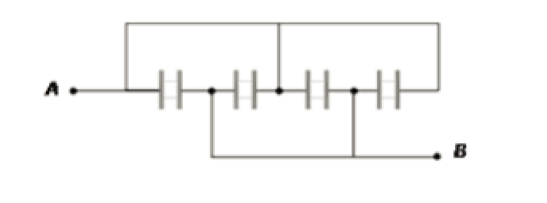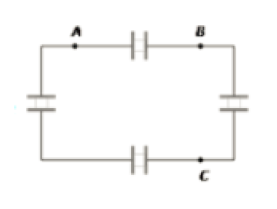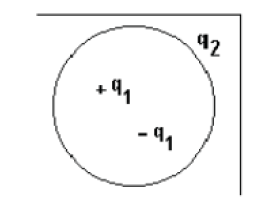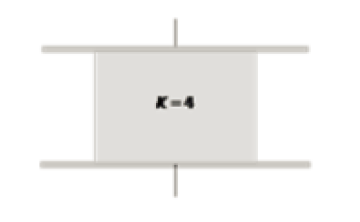## Filters

Sort by :
Clear All
Q
Engineering
137 Views   |

The lines of forces due to charged particles are

• Option 1)

always straight

• Option 2)

always curved

• Option 3)

sometimes curved

• Option 4)

none of the above

As we learnt in  Electric lines of force - The electric field in a region is represented by continious lines field line is an imaginary line along with a Positive test charge will move if left free. -    Line of force due to charge particles are always curved. Option 1) always straight This option is incorrect Option 2) always curved This option is correct Option 3) sometimes curved This...
Engineering
819 Views   |

The force between two charges is 120N. If the distance between the charges is doubled, then the force will be

• Option 1)

60N

• Option 2)

30N

• Option 3)

40N

• Option 4)

15N

As we learnt in  Coulombic force - - wherein K - proportionality Constant  Q1 and Q2 are two Point charge     Option 1) 60N This option is incorrect Option 2) 30N This option is correct Option 3) 40N This option is incorrect Option 4) 15N This option is incorrect
Engineering
144 Views   |

The comb (rubbed on dry hair) attract small pieces of papers and this process is due to

• Option 1)

Paper is a good conductor

• Option 2)

Paper is an insulator

• Option 3)

Both a and b

• Option 4)

None of these

As we learnt in Methods of charging 1. By friction - When two bodies rub together both positive and negative charges in equal amounts appears simultaneously due to transfer of electron. -    When comb is rubbed on dry hair, it will deposit charge due to friction and this will create polarisation in the paper and hence it will exert force on paper. Option 1) Paper is a good conductor This...
Engineering
135 Views   |

The ability of charged bodies to exert force on one another is attributed to the existence of

• Option 1)

electrons

• Option 2)

protons

• Option 3)

neutrons

• Option 4)

electric field

As we learnt in  Electric Field - The space around a charge in which another charged particle experiences a force is said to have electric field in it. - wherein   One charge exert force on another charge due to p electric field produced by one charge and this field will exert force on another charge. Option 1) electrons This option is incorrect Option 2) protons This option is...
Engineering
92 Views   |

If we place an electric dipole S in a uniform electric field W. The dipole has minimum potential energy when angle between S and W in degrees will be

• Option 1)

30

• Option 2)

70

• Option 3)

90

• Option 4)

Zero

As we learnt in  Work done in rotation - -   Energy of dipole is  Energy is minimum  When  Option 1) 30 This option is incorrect Option 2) 70 This option is incorrect Option 3) 90 This option is incorrect Option 4) Zero This option is correct
Engineering
464 Views   |

If we merged 125 spherical mercury droplets having 2.5 V potential of each mercury droplet. What will be the potential of entire droplets will be?

• Option 1)

0.1 V

• Option 2)

1.0 V

• Option 3)

62.5 V

• Option 4)

4 V

v' = n v

given, n= 125

therefore, 125 = 25

that is, v' = 25 * 2.5 = 62.5

Engineering
333 Views   |

If a person brings an electron towards another electron then the change in potential energy of the entire system will be

• Option 1)

Increases

• Option 2)

Half

• Option 3)

Decreases

• Option 4)

Reduces to zero

As we learnt in Potential Energy Of System Of two Charge -          - wherein     If bring two electrons together then r decreases, U increases. Option 1) Increases This option is correct Option 2) Half This option is incorrect Option 3) Decreases This option is incorrect Option 4) Reduces to zero This option is incorrect
Engineering
468 Views   |

If a charge of 1 micro coulomb is divided into 2 parts in ratio 2:3 and placed 1 m apart in the vacuum. What will be the electric forces acting between in Newton?

• Option 1)

0.00216

• Option 2)

216.0

• Option 3)

0.216

• Option 4)

0.0216

As we learnt in  Coulombic force - - wherein K - proportionality Constant  Q1 and Q2 are two Point charge    Charges are                Option 1) 0.00216 This option is correct Option 2) 216.0 This option is incorrect Option 3) 0.216 This option is incorrect Option 4) 0.0216 This option is incorrect
Engineering
490 Views   |

If 4 point charges each +c is placed on the circumference of a circle of the diameter 2S if such a way that position of these 4 charges form a square on the circumference of the circle. Then, what is the potential at the center of the circle?

• Option 1)

4c / S

• Option 2)

0

• Option 3)

3c/S

• Option 4)

S / 4c

As we learnt in  Potential of a System of Charge - - wherein   Potential at centre,  Option 1) 4c / S Correct Option 2) 0 Incorrect Option 3) 3c/S Incorrect Option 4) S / 4c Incorrect
Engineering
254 Views   |

Four condensers are joined as shown in the adjoining figure. The capacity of each is4μF. The equivalent capacity between the points A and B will be• Option 1)

8μF

• Option 2)

16μF

• Option 3)

20μF

• Option 4)

24μF

As we learnt in Parallel Grouping - - wherein    All four are in parallel. Option 1) 8μF This option is incorrect Option 2) 16μF This option is correct Option 3) 20μF This option is incorrect Option 4) 24μF This option is incorrect
Engineering
438 Views   |

Four capacitors of each of capacity 3μFare connected as shown in the adjoining figure. The ratio of equivalent capacitance between A and B and between A and C will be• Option 1)

4:3

• Option 2)

3:4

• Option 3)

2:3

• Option 4)

3:2

its wrong the option should be first one

Engineering
122 Views   |

Find the distance of the point from the charge of potential and field strength at that point is 600 V and 200 N/C.

• Option 1)

6 m

• Option 2)

7 m

• Option 3)

3.5 m

• Option 4)

3 m

As we learnt in  Electric field and potential Due to various charge Distribution i) Point Charge - Electric field and Potential at Point P due to a Point charge Q.     ,      - wherein     Option 1) 6 m Incorrect Option 2) 7 m Incorrect Option 3) 3.5 m Incorrect Option 4) 3 m Correct
Engineering
671 Views   |

Consider the charge configuration and a spherical Gaussian surface as shown in the figure. When calculating the flux of the electric field over the spherical surface, the electric field will be caused due to• Option 1)

• Option 2)

all the charges

• Option 3)

only the +ve charges

• Option 4)

As we learnt in Gauss's Law - Total flux linked with a closed surface called Gaussian surface. Formula:   - wherein No need to be a real physical surface. Qenc - charge enclosed by closed surface.    When we calculate flux we take only charges inside the Gaussian surface. But the electric field is caused by all the charges present. Option 1) This option is incorrect Option 2) all the...
Engineering
371 Views   |

Consider a parallel plate capacitor of 8μF (micro-farad) with air filled in the gap between the plates. Now one half of the space between the plates is filled with a dielectric of dielectric constant 4, as shown in the figure. The capacity of the capacitor changes to• Option 1)

8μF

• Option 2)

16μF

• Option 3)

20μF

• Option 4)

24μF

As we learnt in  Parallel Grouping - - wherein   After inserting dielectric, we will have Capacitance,                                                      Option 1) 8μF This option is incorrect Option 2) 16μF This option is incorrect Option 3) 20μF This option is correct Option 4) 24μF This option is incorrect
Engineering
146 Views   |

A parallel plate capacitor is charged and the charging battery is then disconnected. If the plates of the capacitor are moved further apart by means of insulating handles, then

• Option 1)

the charge on the capacitor increases

• Option 2)

the voltage across the plates decreases

• Option 3)

the capacitance increases

• Option 4)

the electrostatic energy stored in the capacitor increases

As we learnt in  Parallel Plate Capacitor - - wherein Area - A seperation between two plates.    and Energy Stored - -    If we move the plates apart, its capacitance,    will decrease. It will have impact on its potential which will decrease but charge will remain same.     will increase as Q is constant and C decreases.   Option 1) the charge on the capacitor increases This option is...
Engineering
91 Views   |

A capacitor stores 0.24 C at 10V . Its capacitance is

• Option 1)

0.024F

• Option 2)

2.4F

• Option 3)

0.12F

• Option 4)

0.8F

As we learnt in Capacitance of Conductor - - wherein C - Capacity or capacitance of conductor  V - Potential.     Option 1) 0.024F This option is correct Option 2) 2.4F This option is incorrect Option 3) 0.12F This option is incorrect Option 4) 0.8F This option is incorrect
Exams
Articles
Questions